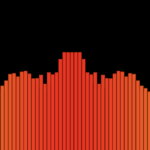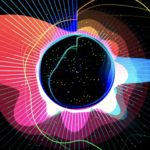# Audio Wave

Introduction:
For this assignment, I wanted to explore how to use sound in p5js and learn how to use object-oriented programming creatively. While exploring my options, I found some ways to do audio visualization, which I found pretty interesting, and then I went on to explore.

Coding and Process
First of all, I learned how to import sounds, use them, and their different functions. Then I familiarized myself with the syntax of object-oriented programming and how to use the classes in p5js. For practice, I tried to make bouncy particles and add features. So, after learning everything, I explored different ways to create audio visualization and took inspiration from the following pictures to recreate an audio-responsive wave with particles around it. Then I learned to use FFT objects and the different functions related to them. It took me time to understand how this works, but the result paid off, I believe.The part of the code I am proud of is the waveform and how the particles respond to the audio.

```let wave = fft.waveform(); //Returns an array of amplitude values (between -1.0 and +1.0)
stroke(random(255), random(255), random(255));

fft.analyze();
amp = fft.getEnergy(20, 200); //Returns the amount of volume at a specific frequency, using which we could use to control the behaviour of particle and thier responsiveness

strokeWeight(2);
beginShape();
//to built the waveform so we use the applitudes and that we got before and then use this to make the wave
for (let i = 0; i <= width; i++) {
let index = floor(map(i, 0, width, 0, wave.length / 2));
let x = i;
let y = wave[index] * 100 + height / 2;
vertex(x, y);
}
endShape();

let p = new Particle(2);
particles.push(p);

//creates the particle around the wave form
for (let i = 0; i < particles.length; i++) {
if (!particles[i].edge()) {
particles[i].move(amp > 100);
particles[i].show();
} else {
particles.splice(i, 1); //if the particle is out of the space then delete it from the array
}```

Final Project

Here’s my code!

Reflection and future improvements

I really enjoyed doing this assignment and exploring my options. The project could be improved by adding more layers or by making a circular waveform and adding more details.